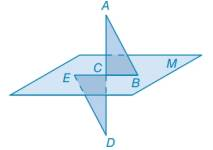Chapter 3.1, Problem 39E### Elementary Geometry for College St...

6th Edition
Daniel C. Alexander + 1 other
ISBN: 9781285195698

#### Solutions

Chapter
Section### Elementary Geometry for College St...

6th Edition
Daniel C. Alexander + 1 other
ISBN: 9781285195698
Textbook Problem
1 views

# In Exercises 39 to 40, complete each proof. Given: Plane M C is the midpoint of E B ¯ A D ¯ ⊥ B E ¯ and A B ¯ ∥ E D ¯ Prove: Δ A B C ≅ Δ D E CTo determine

To prove:

ΔABC is congruent to ΔDEC.

Explanation

Approach:

If two parallel lines are cut by a transversal, then the two alternate interior angles formed are congruent.

Given:

The triangles ΔABC and ΔDEC are shown in the figure below,

Figure (1)

From figure (1),

C is the midpoint of EB¯

And,

AB¯ED¯

Calculation:

Consider the triangles,

ΔABC and ΔDEC

From the given data,

C is the midpoint of EB¯. So, BC¯=CE¯

Since, the equal sides of the triangles are congruent

### Still sussing out bartleby?

Check out a sample textbook solution.

See a sample solution

#### The Solution to Your Study Problems

Bartleby provides explanations to thousands of textbook problems written by our experts, many with advanced degrees!

Get Started

#### Find more solutions based on key concepts